# Merging Sorted Arrays:

## Preparation for Merge Sort Algorithm (Javascript)

To understand better how Merge Sort Algorithm works it’s a good idea to start by learning how to implement a function that merges two arrays.

## Time complexity :

Because we have to take two different arrays, those can vary in length so the time complexity will be: O(n +m)

## How it works:

This function is going to take to arrays sorted in the same way and compare both of them. In order to compare them we’ll create two variables (i and j)to take care of the indexes of each array and also an array to store the results. Then we’ll iterate over them using a while…

# Sorting Algorithms: Insertion Sort

## (Javascript Implementation)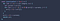Insertion Sort Algorithm

## Time complexity:

Worst case: O(n²)

## How it works :

Insertion sort works a little bit different than Selection Sort and Bubble Sort.

What Insertion sort does in order to sort the array is creating an imaginary slice of the left part that is always sorted.

This could sound a little bit complex but is very easy. Let’s say that we consider the first element sorted, so that would be the sorted slice so far.
Once we compare it with the second element we’ll figure out if the second element is sorted or not compared with the first element.

If the second element is greater than the…

# Sorting Algorithms : Selection Sort

## (Javascript implementation)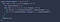Selection Sort Algorithm

## Time Complexity :

Worst Case : O(n²)
It could be slightly better than “Bubble Sort” in case that you want to minimize the swaps.

## How it works :

Contrary to Bubble Sort, this algorithm will start sorting the elements towards the beginning of the array instead of the end.

To accomplish that it needs to create a variable where it will be storing the lowest value and comparing with the rest of the elements.

If during the iteration finds a lower value, it will update our variable so when the iteration is done, the lowest value will swap with the first index of that iteration.

In order…

# Sorting Algorithms : Bubble Sort

## (Javascript implementation)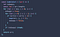Bubble Sort Algorithm

## Time Complexity :

Worst Case: O (n²)
If we use our optimized version and the given array is sorted the best case would be O(n).

## How it works :

Bubble Sort it’s going to loop through the array and compare two adjacent elements, so it can swap them in case one is bigger than the other.
It will repeat this operation until the array is sorted.

# Naive String Searching Algorithm

## (Javascript Implementation)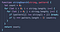Naive String Searching Algorithm

## Time complexity:

Worst Case: O(m*n)

## How it works:

We’re going to create a function with a parameter for the givenString and a parameter for the pattern we’re looking for.

First let’s create a counter to count how many matches do we find.

Next, in order to compare the two strings, we need to create a loop to take care of all the indexes of the givenString and also an inner loop to compare the indexes.

While we are in the index 0 of the outer loop, the inner loop will check if the elements contained on the index 0 of the givenString and pattern are…

# Searching Algorithms: Binary Search

## (Javascript implementation)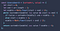Binary Search Algorithm

This search algorithm works on the principle of divide and conquer. For this algorithm to work properly, there is a big caveat: the data collection must be sorted.

## Time complexity:

• Worst and Average Case: O(log n)
• Best Case: O(1)

# How Binary Search Works?

Binary search is going to compare the element we are looking for with the middle element of the collection. If there is a match, it will return the index of the middle item. If the middle item is shorter than the item we’re looking for, we forget about the sub-array from the middle to the right, and repeat the operation searching for the…

# Searching Algorithms: Linear Search (Javascript)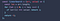Linear Search implementation

# Linear Search :

Linear search is probably the easiest searching algorithm out there. It’s going to check one by one all the elements of an array in a sequential order.

## Time complexity

As “n” grows, the average amount of time it takes will grow. If we have an array with a million elements it is going to search one by one until it finds the passed value.

Best case O(1).
Even if it’s pretty rare, depending on the size of the data. The Best Case would be find the thing we’re looking for right away.

Worst Case is O(n) In case the element we’re searching…## Edur

Computer Science and Software Engineering